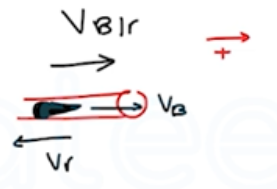Need Help?

Subscribe to Physics 1

###### \${selected_topic_name}
• Notes

$\begin{array}{l}{\text { A } 68.5-\mathrm{kg} \text { astronaut is doing a repair in space on the orbit- }} \\ {\text { ing space station. She throws a } 2.25-\mathrm{kg} \text { tool away from her at }} \\ {3.20 \mathrm{m} / \mathrm{s} \text { relative to the space station. With what speed and in }} \\ {\text { what direction will she begin to move? }}\end{array}$

$m_{A}=68.5 \mathrm{kg} \quad, \quad m_B=2.25 \mathrm{kg}$

$V_{A 1x}=V_{B 1x}=0 \quad, \quad V_{B 2 x}=3.2 \mathrm{m/s}$

$P_{1 x}=P_{2 x} \rightarrow P_{1 x}=m_{A}v_{A1} x+m_{B} v_{B x 1}=0$

$P_{2}=m_{A} v_{A x 2}+m_{B} v_{B x{2}}=0$

$m_{A} v_{A} x_ 2=- m_{B}v_B x_{2}$

$v_{A x 2}=-\frac{m_{B} v_{B}x_2}{m_{A}}=-\frac{(2.25)(3.2)}{(68.5)}=-0 .105 m / s$

$\text { opposite direct. }$

$\begin{array}{l}{\text { The expanding gases that leave the muzzle of a rifle also }} \\ {\text { contribute to the recoil. A } 30 \text { -caliber bullet has mass } 0.00720 \mathrm{kg}} \\ {\text { and a speed of } 601 \mathrm{m} / \mathrm{s} \text { relative to the muzzle when fired from a }} \\ {\text { rifle that has mass } 2.80 \mathrm{kg} \text { . The loosely held rifle recoils at a speed }} \\ {\text { of } 1.85 \mathrm{m} / \mathrm{s} \text { relative to the earth. Find the momentum of the pro- }} \\ {\text { pellant gases in a coordinate system attached to the earth as they }} \\ {\text { leave the muzzle of the rifle. }}\end{array}$$m_{B}=0.0072 \mathrm{kg}$

$m_{r}=2.8 \mathrm{kg}$

$V_{r}=-1.85 \mathrm{m/s}$

$V_{B / r}=601 \mathrm{m/s}$

$v_{b / r}=v_{b}-v_{r} \longrightarrow v_{b}=v_{b / r}+v_{r}$

$=601-1.85=599 \mathrm{m/s}$

$P_{x{1}}=P_{x 2}$

$P_{g x} \quad \longrightarrow \quad P_{r x}+P_{Bx}+P_{gx}=0$

$P_{G}=-P_{rx} -P_{B x}=-(2.8*-1.85)-(0.0072)(599)$

$∴ P_{G}=0.87 \mathrm{kg} \cdot \mathrm{m} / \mathrm{s}$

$\begin{array}{l}{\text { You are standing on a sheet of ice that covers the football }} \\ {\text { stadium parking lot in Buffalo; there is negligible friction between }} \\ {\text { your feet and the ice. A friend throws you a } 0.400-\mathrm{kg} \text { ball that is }} \\ {\text { traveling horizontally at } 10.0 \mathrm{m} / \mathrm{s} \text { . Your mass is } 70.0 \mathrm{kg} \text { . (a) If you }} \\ {\text { catch the ball, with what speed do you and the ball move after- }} \\ {\text { ward? (b) If the ball hits you and bounces off your chest, so after- }} \\ {\text { ward it is moving horizontally at } 8.0 \mathrm{m} / \mathrm{s} \text { in the opposite direction, }} \\ {\text { what is your speed after the collision? }}\end{array}$

$m_{A}=70 kg \quad, \quad m_{B}=0.4 k g$

$V_{A{1}}=0 \quad V_{B 1}=10 \mathrm{m} / \mathrm{s}$

$V _{B2}=V _{A2}=V_{X 2}$

$P_{x{1}}=P_{x 2} \longrightarrow m_{A} v_{A{1}}+m_{B} v_{B 1}=m_{A} v_{A 2 x}+m_{B} v_{B 2 x}$

$\longrightarrow m_{B} v_{B 1 x}=m_ A V_{x 2}+m_{B} v_{x2} \rightarrow m_{B} v_{B 1 x}=v_{x{2}}\left(m_{A}+m_{B}\right)$

$\rightarrow V_{x 2}=\frac{m_{B} v_{B X 1}}{m_ A+m_{B}}=\frac{0.4* 10}{0.4+70}=0.0568 \mathrm{m} / \mathrm{s}$

$V_{BX 2} \neq V_{A X 2} \ , V_{B X 2}=-8 \mathrm{m/s}$

$0.4(10)=(70) V_{A 2}+0.4(-8) \rightarrow V_{A 2}=\frac{0.4(10)+0.4(8)}{70}$

$=0.103 \mathrm{m/s}$